# US9384371B2 - Compact CMOS current-mode analog multifunction circuit - Google Patents

## Info

Publication number
US9384371B2
US9384371B2 US14/526,474 US201414526474A US9384371B2 US 9384371 B2 US9384371 B2 US 9384371B2 US 201414526474 A US201414526474 A US 201414526474A US 9384371 B2 US9384371 B2 US 9384371B2
Authority
US
United States
Prior art keywords
current
currents
gain
characterized
in1
Prior art date
Legal status (The legal status is an assumption and is not a legal conclusion. Google has not performed a legal analysis and makes no representation as to the accuracy of the status listed.)
Active, expires
Application number
US14/526,474
Other versions
US20160117527A1 (en
Inventor
Eyas Saleh Al-Suhaibani
Munir A. Al-Absi
Current Assignee (The listed assignees may be inaccurate. Google has not performed a legal analysis and makes no representation or warranty as to the accuracy of the list.)
King Fahd University of Petroleum and Minerals
Original Assignee
King Fahd University of Petroleum and Minerals
Priority date (The priority date is an assumption and is not a legal conclusion. Google has not performed a legal analysis and makes no representation as to the accuracy of the date listed.)
Filing date
Publication date
Application filed by King Fahd University of Petroleum and Minerals filed Critical King Fahd University of Petroleum and Minerals
Priority to US14/526,474 priority Critical patent/US9384371B2/en
Assigned to KING FAHD UNIVERSITY OF PETROLEUM AND MINERALS reassignment KING FAHD UNIVERSITY OF PETROLEUM AND MINERALS ASSIGNMENT OF ASSIGNORS INTEREST (SEE DOCUMENT FOR DETAILS). Assignors: AL-ABSI, MUNIR A., DR., AL-SUHAIBANI, EYAS SALEH, MR.
Publication of US20160117527A1 publication Critical patent/US20160117527A1/en
Application granted granted Critical
Publication of US9384371B2 publication Critical patent/US9384371B2/en
Application status is Active legal-status Critical

• 239000004065 semiconductor Substances 0 claims 2
• 238000009740 moulding (composite fabrication) Methods 0 abstract 1
• 238000004088 simulation Methods 0 description 10
• 238000005516 engineering processes Methods 0 description 3
• 230000000051 modifying Effects 0 description 3
• 230000004044 response Effects 0 description 2
• 239000000969 carrier Substances 0 description 1
• 230000000875 corresponding Effects 0 description 1
• 230000001965 increased Effects 0 description 1
• 238000000034 methods Methods 0 description 1
• 238000005365 production Methods 0 description 1
• 230000001629 suppression Effects 0 description 1

## Images

•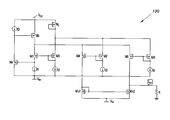•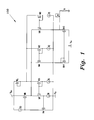•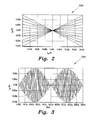•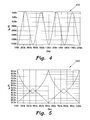•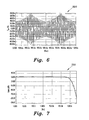•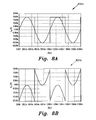•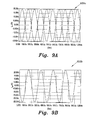•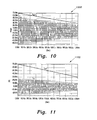## Classifications

• GPHYSICS
• G06COMPUTING; CALCULATING; COUNTING
• G06GANALOGUE COMPUTERS
• G06G7/00Devices in which the computing operation is performed by varying electric or magnetic quantities
• G06G7/12Arrangements for performing computing operations, e.g. operational amplifiers
• G06G7/16Arrangements for performing computing operations, e.g. operational amplifiers for multiplication or division

## Abstract

The compact CMOS current-mode analog multifunction circuit is based on an implementation using MOSFETs operating in a sub-threshold region and forming two overlapping translinear loops capable of performing multiplication, division, controllable gain current amplifier, current mode differential amplifier, and differential-input single-output current amplifier.

## Description

BACKGROUND OF THE INVENTION

1. Field of the Invention

The present invention relates to electronic function circuits, and particularly to a compact CMOS current-mode analog multifunction circuit operating in the weak inversion region.

2. Description of the Related Art

Low power and low voltage current-mode analog computational circuits got an increasing interest lately especially as CMOS fabrication technology advances. Using current-mode circuits, many functions can be design with less number of components compared to the voltage-mode counterpart. Nowadays, low voltage and ultra-low power analog circuits are becoming mandatory in battery powered applications. CMOS circuits operating in subthreshold region can be used to realize a low voltage and low power circuits. Multiplication and division are important analog signal processing functions. Having such circuits that consumed very low power is a great advantage. That is because they can be used in portable devices especially devices that are to be used in remote areas. There are many approaches reported in the literature to design multiplier, squaring, square rooter and divider circuits.

Although there are many proposed circuits for current-mode analog multipliers and dividers, many of them are designed to work only as multipliers and dividers. Having a single circuit that can perform more than one function is preferable.

Thus, a compact CMOS current-mode analog multifunction circuit solving the aforementioned problems is desired.

SUMMARY OF THE INVENTION

The compact CMOS current-mode analog multifunction circuit is based on an implementation using MOSFETs operating in a sub-threshold region to form translinear loops.

These and other features of the present invention will become readily apparent upon further review of the following specification and drawings.

BRIEF DESCRIPTION OF THE DRAWINGS

FIG. 1 is a circuit diagram of the CMOS current-mode analog multifunction circuit according to the present invention.

FIG. 2 is a multiplier DC transfer plot of the CMOS current-mode analog multifunction circuit according to the present invention.

FIG. 3 is a plot of a multiplier as DSBSC AM modulator transfer of the CMOS current-mode analog multifunction circuit according to the present invention.

FIG. 4 is a plot of a simulation result for squaring function of the CMOS current-mode analog multifunction circuit according to the present invention.

FIG. 5 is a plot of a simulation result for divide function of the CMOS current-mode analog multifunction circuit according to the present invention.

FIG. 6 is a plot showing the result of dividing a sinusoidal signal by a triangular signal using the CMOS current-mode analog multifunction circuit according to the present invention.

FIG. 7 is a plot of the frequency response of the CMOS current-mode analog multifunction circuit according to the present invention.

FIG. 8A is a plot of the input signal of the differential amplifier of the CMOS current-mode analog multifunction circuit according to the present invention.

FIG. 8B is a plot of the output signal of the differential amplifier of the CMOS current-Mode Analog multifunction circuit according to the present invention.

FIG. 9A is another plot of the input signal of a differential input single output amplifier of the CMOS current-Mode Analog multifunction circuit according to the present invention.

FIG. 9B is another plot of the output signal of a differential input single output amplifier of the CMOS current-Mode Analog multifunction circuit according to the present invention.

FIG. 10 is a plot of the gain change under a differential input single output amplifier configuration of the CMOS current-Mode Analog multifunction circuit according to the present invention.

FIG. 11 is a plot of the gain change under a controllable gain current amplifier configuration of the CMOS current-Mode Analog multifunction circuit according to the present invention.

Similar reference characters denote corresponding features consistently throughout the attached drawings.

DETAILED DESCRIPTION OF THE PREFERRED EMBODIMENTS

The compact CMOS current-mode analog multifunction circuit 100 (shown in FIG. 1) is based on an implementation using MOSFETs operating in a sub-threshold region to form translinear loops. The circuit 100 consists of six-matched transistors that form two overlapping translinear loops. In circuit 100, the first transistor loop is formed by the transistors M1, M2, M3, and M4. The second translinear loop is formed by the transistors M1, M2, M5, and M6. By modifying the input currents, the present circuit can be used to implement many functions. With reference to FIG. 1, the equation around the translinear loop formed by transistors M1, M2, M3 and M4 is given by:
I 1 I 2 =I 3 I 4.  (1)

And the translinear loop formed by transistors M1, M2, M5 and M6 is given by:
I 1 I 5 =I 3 I 6,  (2)
where, Ii is the drain current for the transistor Mi. Let I4 be the output of the first translinear loop and I6 be the output of the second translinear loop. Then, the difference between the two output currents is considered to be the output of the present circuit. That is:

$I out = I 4 - I 6 = I 1 ⁡ ( I 2 - I 5 ) I 3 ( 3 )$
Equation (3) is used to produce different functions as described below.

The present circuit can be used as a four-quadrant multiplier if the currents I1, I2, I3, and I5 are set to the value shown in Table 1.

TABLE 1 Four-quadrant multiplier Four-quadrant multiplier I1 = I0 + iin1 I2 = I0 + iin2 I3 = I0 I5 = I0 − iin2

The currents iin1 and iin2 are AC input signals and they are shifted by a DC quantity, I0. Substituting the above values into equation (3), it is easy to show that:

$I 4 - I 6 = 2 ⁢ i i ⁢ ⁢ n ⁢ ⁢ 2 + 2 ⁢ i i ⁢ ⁢ n ⁢ ⁢ 1 ⁢ i i ⁢ ⁢ n ⁢ ⁢ 2 I 0 ( 4 )$
if the 2iin2 term is subtracted from equation 4, a four-quadrant multiplier can be achieved, and the output current is given by:

$I out = 2 ⁢ i i ⁢ ⁢ n ⁢ ⁢ 1 ⁢ i i ⁢ ⁢ n ⁢ ⁢ 2 I 0 ( 5 )$
It is very clear that this four-quadrant multiplier can be used as a squaring function if iin1=iin2=iin, the output current is given by:

$I out = 2 ⁢ i i ⁢ ⁢ n 2 I 0 ( 6 )$

The present circuit can also be used as a two-quadrant divider. With reference to equation (3) the term (I2−I5) is set to be a pure AC signal, this will be the dividend and the divisor will be I3. In other words, let the currents I1, I2, I3, and I5 be set to the values shown in Table 2.

TABLE 2 Two-quadrant divider Two-quadrant divider I1 = IGain I2 = I0 + iin1 I3 = Iin2 I5 = I0 − iin1

Then the output will be given by:

$I out = I 4 - I 6 = 2 ⁢ I Gain ⁢ i i ⁢ ⁢ n ⁢ ⁢ 1 I i ⁢ ⁢ n ⁢ ⁢ 2 ( 7 )$
It is clear that equation (7) implements a divide function with controllable gain.

Referring to equation (3), the present circuit can also be used as a current mode differential amplifier. Consider the values shown in Table 3 for the translinear loop currents:

TABLE 3 Current mode differential amplifier Current Mode Differential Amplifier I1 = IGain1 I2 = I0 + iin1 I3 = IGain2 I5 = I0 − iin2

The currents I1, and I3 can be used to control the gain of the differential amplifier, the output will be given by:

$I out = I 4 - I 6 = I Gain ⁢ ⁢ 1 ⁡ ( i i ⁢ ⁢ n ⁢ ⁢ 1 - i i ⁢ ⁢ n ⁢ ⁢ 2 ) I Gain ⁢ ⁢ 2 ( 8 )$

Differential-input-single-Output current amplifier is achieved, if the translinear loop currents are set to the values shown in Table 4.

TABLE 4 Differential input single output current amplifier Differential input single output current amplifier I1 = IGain1 I2 = I0 + iin1 I3 = IGain2 I5 = I0 − iin1

The output is given by:

$I out = I 4 - I 6 = 2 ⁢ I Gain ⁢ ⁢ 1 ⁡ ( i i ⁢ ⁢ n ⁢ ⁢ 1 ) I Gain ⁢ ⁢ 2 ( 9 )$
It is clear that equation (9), implements a differential input single output amplifier with flexible gain control using currents IGain1 and IGain2.

If one of the inputs (e.g., iin2) in the differential-input single-output current amplifier is set to zero, then a controllable gain current amplifier is obtained. The translinear loop currents are set according values shown in Table 5.

TABLE 5 Controllable gain current amplifier Controllable gain current amplifier I1 = IGain1 I2 = I0 + iin1 I3 = IGain2 I5 = I0

Following the same procedure, the output will be as follows:

$I out = I 4 - I 6 = I Gain ⁢ ⁢ 1 ⁡ ( i i ⁢ ⁢ n ⁢ ⁢ 1 ) I Gain ⁢ ⁢ 2 ( 10 )$

Tanner T-spice with 0.35 μm CMOS technology is used to confirm the functionality of the proposed circuit. Table 6 shows the aspect ratios for all transistors used in the simulation. The circuit is operated from ±0.75 DC supply, the input currents for the multiplier are swept from −20 nA to 20 nA. Simulation result shown in plot 200 of FIG. 2 confirms the functionality of the multiplier function.

TABLE 6 Transistor aspect ratios of the present circuit. Transistor Ma and Mb Mc M1-M6 Mn1-Mn2 W (μm)/L (μm) 50/0.4 10/0.4 9.2/5 1.5/4.5

Application of the multiplier as a double sideband suppressed carrier (DSBSC) modulator was simulated. The simulation result is shown in plot 300 of FIG. 3.

Plot 400 of FIG. 4 shows the result of a squaring function when an input sinusoidal signal having frequency of 1 kHz and an amplitude of 20 nA is applied. Simulation results confirm the functionality of the circuit.

Plot 500 of FIG. 5 shows the result of using the present circuit as a two quadrant divider to divide a DC signal by a triangular signal. Also, plot 600 of FIG. 6 shows the result of dividing a sinusoidal signal by a triangular signal.

Simulation for frequency response was carried out for the multiply function. Simulation result shown in plot 700 of FIG. 7 indicates that the −3 dB frequency is around 1 MHz. The Total Harmonics Distortion (THD) of the proposed circuit was calculated by applying a sine wave signal with frequency of 1 kHz and then calculating the ratio of the power of the 1000 harmonics to the power of the fundamental frequency. The THD came to be 0.13%.

Plots 800 a and 800 b of FIGS. 8A and 8B, respectively, show the input (FIG. 8A) and output (FIG. 8B) current signals when using the circuit as a difference amplifier. It is clear that the circuit is subtracting the square signal from the sinusoidal one.

The circuit 100 was also simulated for a differential-input single-output current amplifier. The differential input and output signal are shown in plots 900 a and 900 b of FIGS. 9A and 9B, respectively.

Plot 1000 of FIG. 10 shows the simulation result for the output current when IGain1 is varied from 70 nA to 30 nA. It is clear that the gain of the output changes accordingly.

Plot 1100 of FIG. 11 shows the simulation result when using the present circuit as a controllable gain current amplifier. The input is a sinusoidal signal and the gain is controlled by varying IGain1 from 70 nA to 30 nA.

The performance of the present design was compared with previously published work in the open literature and is summarized in Table 7.

TABLE 7 Performance comparison M. Gravati, A, Mahmoudi, M. Valle, A. Khoei and G. Ferri, K. Tanno, KH, Hadidi. N. Guerrini, Y. Sugahara, Reference (2007) L. Reyes, H. Tamura. This work Year 2007 2005 2011 2013 Power Supply 2 V 2 V 1 V ±0.75 V Technology 0.35 μm 0.35 μm 0.18 μm 0.35 μm Bandwidth <10 MHz 200 kHz 768 kHz 1 MHz THD  <1% 0.90% 1.30% 0.14% Linearity error 2.8%   5% 0.88%  0.5% Power 9 μW 5.5 μW 1.12 μW 1.4 μW Consumption Functions Multiply and Multiply Multiply Multiply, divide divide, and three different types of amplifiers

It is clear from the table that the present design has a better performance in terms of power consumption, linearity error, and THD, and the number of functions it can implement compared to most of the related designs. Also, its bandwidth is better than most of the other published works. The present circuit 100 implements many functions with less number of transistors compared to other designs.

The present invention provides a new current-mode analog multi-function circuit capable of performing multiplication, division, controllable gain current amplifier, current mode differential amplifier, and differential-input single-output current amplifier. The circuit is compact and can be a useful building block in analog signal processing applications.

It is to be understood that the present invention is not limited to the embodiments described above, but encompasses any and all embodiments within the scope of the following claims.

## Claims (8)

We claim:
1. A compact CMOS current-mode analog multifunction circuit, comprising:
a first translinear loop comprised of metal-oxide-semiconductor field-effect transistors (MOSFETs) M1, M2, M3, and M4;
a second translinear loop comprised of metal-oxide-semiconductor field-effect transistors (MOSFETs) M1, M2, M5, and M6, first and second translinear loops overlapping and configured to operate in a sub-threshold (weak inversion) region, input iin1 of the first translinear loop consisting of at least one drain current of the first translinear loop MOSFETS, input iin2 of the second translinear loop consisting of at least one drain current of the second translinear loop MOSFETS;
currents of the first translinear loop being characterized by the relation,

I 1 I 2 =I 3 I 4,
where Ii is the drain current for the transistor Mi and I4 is the output of the first translinear loop;
currents of the second translinear loop being characterized by the relation,

I 1 I 5 =I 3 I 6,
where Ii is the drain current for the transistor Mi, and I6 is the output of the second translinear loop;
wherein an output current of the current-mode analog multifunction circuit is a difference between the outputs of the first and second translinear loops.
2. The compact CMOS current-mode analog multifunction circuit according to claim 1, further comprising:
currents in the circuit characterized by the relations,

I 1 =I 0 +i in1

I 2 =I 0 +i in2

I 3 =I 0

and

I 5 =I 0 −i in2; and
wherein the input currents iin1 and iin2 are AC input signals shifted by a DC quantity I0, resulting in a four quadrant multiplication of the input currents at the output of the current-mode analog multifunction circuit after subtracting 2iin2 from the output current, said currents being characterized by the relations,
$I 4 - I 6 = 2 ⁢ i i ⁢ ⁢ n ⁢ ⁢ 2 + 2 ⁢ i i ⁢ ⁢ n ⁢ ⁢ 1 ⁢ i i ⁢ ⁢ n ⁢ ⁢ 2 I 0$ $I out = 2 ⁢ i i ⁢ ⁢ n ⁢ ⁢ 1 ⁢ i i ⁢ ⁢ n ⁢ ⁢ 2 I 0 .$
3. The compact CMOS current-mode analog multifunction circuit according to claim 2, further comprising:
currents in the circuit characterized by the relation,

i in1 =i in2 =i in; and
wherein the output current is characterized by the relation,
$I out = 2 ⁢ i i ⁢ ⁢ n 2 I 0 ,$
thereby implementing a squaring function.
4. The compact CMOS current-mode analog multifunction circuit according to claim 1, further comprising:
currents in the circuit characterized by the relations,

I 1 =I Gain,

I 2 =I 0 +i in1,

I 3 =I 2,

I 5 =I 0 −i in1; and
wherein the output current is characterized by the relation,
$I out = I 4 - I 6 = 2 ⁢ I Gain ⁢ i i ⁢ ⁢ n ⁢ ⁢ 1 I i ⁢ ⁢ n ⁢ ⁢ 2 ,$
thereby implementing a divide function with controllable gain.
5. The compact CMOS current-mode analog multifunction circuit according to claim 1, further comprising:
currents in the circuit characterized by the relations,

I 1 =I Gain1,

I 2 =I 0 +i in1,

I 3 =I Gain2,

I 5 =I 0 −i in2; and
wherein the currents I1, and I3 can be used to control the gain of the differential amplifier, the output current being characterized by the relation,
$I out = I 4 - I 6 = I Gain ⁢ ⁢ 1 ⁡ ( i i ⁢ ⁢ n ⁢ ⁢ 1 - i i ⁢ ⁢ n ⁢ ⁢ 2 ) I Gain ⁢ ⁢ 2 ,$
thereby implementing a current mode differential amplifier.
6. The compact CMOS current-mode analog multifunction circuit according to claim 1, further comprising:
currents in the circuit characterized by the relations,

I 1 =I Gain1,

I 2 =I 0 +i in1,

I 3 =I Gain2,

I 5 =I 0 −i in1; and
wherein the output current is characterized by the relation,
$I out = I 4 - I 6 = 2 ⁢ I Gain ⁢ ⁢ 1 ⁡ ( i i ⁢ ⁢ n ⁢ ⁢ 1 ) I Gain ⁢ ⁢ 2 ,$
thereby implementing a differential input single output amplifier with flexible gain control using currents IGain1 and IGain2.
7. The compact CMOS current-mode analog multifunction circuit according to claim 1, further comprising:
currents in the circuit characterized by the relations,

i in2=0,

I 1 =I Gain1,

I 2 =I 0 +i in1,

I 3 =I Gain2,

I 5 =I 0 −i in1; and
wherein the output current is characterized by the relation,
$I out = I 4 - I 6 = I Gain ⁢ ⁢ 1 ⁡ ( i i ⁢ ⁢ n ⁢ ⁢ 1 ) I Gain ⁢ ⁢ 2 ,$
thereby implementing a controllable gain current amplifier.
8. The compact CMOS current-mode analog multifunction circuit according to claim 1, wherein the translinear loop MOSFETs M1 through M6 have an aspect ratio of 9.2μ/5μ.
US14/526,474 2014-10-28 2014-10-28 Compact CMOS current-mode analog multifunction circuit Active 2035-02-28 US9384371B2 (en)

## Priority Applications (1)

Application Number Priority Date Filing Date Title
US14/526,474 US9384371B2 (en) 2014-10-28 2014-10-28 Compact CMOS current-mode analog multifunction circuit

## Applications Claiming Priority (1)

Application Number Priority Date Filing Date Title
US14/526,474 US9384371B2 (en) 2014-10-28 2014-10-28 Compact CMOS current-mode analog multifunction circuit

## Publications (2)

Publication Number Publication Date
US20160117527A1 US20160117527A1 (en) 2016-04-28
US9384371B2 true US9384371B2 (en) 2016-07-05

# Family

## Family Applications (1)

Application Number Title Priority Date Filing Date
US14/526,474 Active 2035-02-28 US9384371B2 (en) 2014-10-28 2014-10-28 Compact CMOS current-mode analog multifunction circuit

## Country Status (1)

US (1) US9384371B2 (en)

## Citations (9)

* Cited by examiner, † Cited by third party
Publication number Priority date Publication date Assignee Title
JPH0554158A (en) 1991-08-27 1993-03-05 Omron Corp Multiplying circuit, division circuit and fixed value calculating circuit to be operated in current mode
US5864255A (en) 1994-06-20 1999-01-26 Unisearch Limited Four quadrant square law analog multiplier using floating gate MOS transitions
US5966040A (en) 1997-09-26 1999-10-12 United Microelectronics Corp. CMOS current-mode four-quadrant analog multiplier
US6201430B1 (en) 1998-12-15 2001-03-13 Kabushiki Kaisha Toshiba Computational circuit
TW449718B (en) 1997-06-28 2001-08-11 Nat Science Council Current-mode four quadrant multiplier and two quadrant divider in subthreshold region
US20110050319A1 (en) 2007-09-03 2011-03-03 Toumaz Technology Limited Multiplier, Mixer, Modulator, Receiver and Transmitter
US8421541B2 (en) 2009-06-27 2013-04-16 Qualcomm Incorporated RF single-ended to differential converter
US8575971B1 (en) * 2011-01-27 2013-11-05 Maxim Integrated Products, Inc. Current mirror and current cancellation circuit
US8610486B1 (en) 2013-07-02 2013-12-17 King Fahd University Of Petroleum And Minerals Current-mode analog computational circuit

## Patent Citations (9)

* Cited by examiner, † Cited by third party
Publication number Priority date Publication date Assignee Title
JPH0554158A (en) 1991-08-27 1993-03-05 Omron Corp Multiplying circuit, division circuit and fixed value calculating circuit to be operated in current mode
US5864255A (en) 1994-06-20 1999-01-26 Unisearch Limited Four quadrant square law analog multiplier using floating gate MOS transitions
TW449718B (en) 1997-06-28 2001-08-11 Nat Science Council Current-mode four quadrant multiplier and two quadrant divider in subthreshold region
US5966040A (en) 1997-09-26 1999-10-12 United Microelectronics Corp. CMOS current-mode four-quadrant analog multiplier
US6201430B1 (en) 1998-12-15 2001-03-13 Kabushiki Kaisha Toshiba Computational circuit
US20110050319A1 (en) 2007-09-03 2011-03-03 Toumaz Technology Limited Multiplier, Mixer, Modulator, Receiver and Transmitter
US8421541B2 (en) 2009-06-27 2013-04-16 Qualcomm Incorporated RF single-ended to differential converter
US8575971B1 (en) * 2011-01-27 2013-11-05 Maxim Integrated Products, Inc. Current mirror and current cancellation circuit
US8610486B1 (en) 2013-07-02 2013-12-17 King Fahd University Of Petroleum And Minerals Current-mode analog computational circuit

## Also Published As

Publication number Publication date
US20160117527A1 (en) 2016-04-28

## Similar Documents

Publication Publication Date Title
Macklin et al. A near–quantum-limited Josephson traveling-wave parametric amplifier
Sansen Distortion in elementary transistor circuits
Prasad et al. Universal current-mode biquad filter using dual output current differencing transconductance amplifier
US5151625A (en) High frequency BiMOS linear V-I converter, voltage multiplier, mixer
Punzenberger et al. A 1.2-V low-power BiCMOS class AB log-domain filter
TW200941928A (en) Low noise and low input capacitance differential MDS LNA
Gravati et al. A novel current-mode very low power analog CMOS four quadrant multiplier
Tangsrirat Novel current-mode and voltage-mode universal biquad filters using single CFTA
Naderi et al. A new high speed and low power four-quadrant CMOS analog multiplier in current mode
US9071197B2 (en) Harmonic rejective passive up converter
US8594609B2 (en) Drain-pumped sub-harmonic mixer for millimeter wave applications
Munoz-Pacheco et al. Frequency limitations in generating multi-scroll chaotic attractors using CFOAs
WO2013091351A1 (en) Transconductance-enhancing passive frequency mixer
Tangsrirat et al. Single-resistance-controlled quadrature oscillator and universal biquad filter using CFOAs
Kumngern et al. Electronically tunable high-input impedance voltage-mode universal biquadratic filter based on simple CMOS OTAs
US20060232355A1 (en) Transmission line transformer
JP3339892B2 (en) Integrated circuits and methods of use thereof
Li A new single MCCCDTA based Wien-bridge oscillator with AGC
Vlassis et al. Differential-voltage attenuator based on floating-gate MOS transistors and its applications
Monsurro et al. Linearization technique for source-degenerated CMOS differential transconductors
Naderi et al. Four-quadrant CMOS analog multiplier based on new current squarer circuit with high-speed
Girling et al. The Q of a capacitor-loaded gyrator
CN1200383C (en) Analog multiply circuit and gain variable amplify circuit
Sagbas et al. Current and voltage transfer function filters using a single active device

## Legal Events

Date Code Title Description
AS Assignment

Owner name: KING FAHD UNIVERSITY OF PETROLEUM AND MINERALS, SA

Free format text: ASSIGNMENT OF ASSIGNORS INTEREST;ASSIGNORS:AL-SUHAIBANI, EYAS SALEH, MR.;AL-ABSI, MUNIR A., DR.;REEL/FRAME:034055/0817

Effective date: 20141014

STCF Information on status: patent grant

Free format text: PATENTED CASE